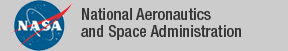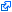### Top File of PVS structures Library

```
top: THEORY
%------------------------------------------------------------------------
%
%  Structures Library
%  ------------------
%
%  Authors:
%
%     Ricky W. Butler        NASA Langley Research Center
%     David Griffioen        CWI (National Research Institute for
%                                 Mathematics and Computer Science
%                                 and KUN (Catholic University Nijmegen),
%                                 the Netherlands.
%     Lee Pike               NASA Langley Research Center
%
%  Version 2.0               9/23/96
%  Version 2.1               12/22/03   no longer needs TYPE+
%  Version 2.2               Merged arrays and bags library into one
%  Version 2.3               Pike greatly extended bags library
%
%  Maintained by:
%
%     Rick Butler            NASA Langley Research Center
%                            R.W.Butler@larc.nasa.gov
%------------------------------------------------------------------------

BEGIN

IMPORTING
% -------------- functions -----------------

const_fun_def,
function_image_bis,        % Extra properties of image and inverse_image
function_inverse_alt_aux,  % Extras for function inverse
function_props_aux,        % inverse_image properties

% -------------- arrays -----------------
top_array,
min_array,       	% defines min function over an array
max_array,       	% defines max function over an array
permutations,    	% permutations defined using arrays
sort_array,      	% defines a sort function over arrays
sort_array_lems, 	% relationship between sort and min and max
array_ops,       	% array operations
majority_array,  	% defines majority function over an array

% -------------- sequences -----------------
top_seq,
seqs,
max_seq,         	% defines max function over an sequence
min_seq,         	% defines min function over a sequence
permutations_seq,	% permutations defined using arrays
majority_seq,    	% defines majority function over finite sequences
bubblesort,      	% bubble sort correctness theorem
sort_seq,        	% defines a sort function over sequences
sort_seq_lems,   	% relationship between sort and min and max
set2seq,             % convert set to sequence
minmax_set2seq,
seq2set,          	% convert sequence to a set
minmax_seq2set,
seq_extras,          % extra-definitions that complements the prelude's finite sequences
seq_pigeon,          % Pigeon hole principle for the prelude's finite sequences

% -------------- bags -----------------
top_bags,
bags,                % fundamental definitions and properties
bags_aux,            % definition of filter, rest, and choose
bags_to_sets,        % converts bags to sets
finite_bags,         % basic definitions and lemmas
finite_bags_lems,    % lemmas need induction
finite_bags_aux,     % lemmas about filter, rest, and choose
finite_bags_inductions,  % induction schemes
bag_filters,         % filtering linearly-ordered bags, pigeonhole,
%   majority pigeonhole results, and overlap
%   existence proof
majority_vote,       % defines a majority vote over finite bags
middle_value_select, % defines middle value selection over finite bags
fault_masking_vote,  % proves an equivalence between majority and middle
%   value selection
finite_bags_minmax,  % defines the minimum and maximum of a finite
%   ordered bag.

% --------------- permutation -------------
permutation,
permutation_ops,

% --------------- lists -------------

more_list_props,
listn,
array2list,
set_as_list,
set_as_list_props,

% --------------- for loops and iterations -------------

for_iterate,
for_examples,
big_ops_nat,

% --------------- arrays -------------
arrays,
arrays_examples,
% --------------- transition systems --------------

runs,
% ----------- Alternate finite sequence (fseq) ----------

fseqs,               % finite sequence using [nat -> T]
fseqs_def,           % finite sequence using [nat -> T] with default parameter
fseqs_ops,           % operations on finite sequence using [nat -> T]
fseqs_ops_def,       % operations on finite sequence using [nat -> T] with default parameter
fseqs_ops_real,      % operations on finite sequence using [nat -> real]
max_fseq,         	% defines max function over an sequence
min_fseq,         	% defines min function over a sequence
permutations_fseq,	% permutations defined using arrays
majority_fseq,    	% defines majority function over finite sequences
sort_fseq,        	% defines a sort function over sequences
sort_fseq_lems,  	% relationship between sort and min and max
fseq2set,         	% convert sequence to a set

Maybe,               % Maybe[T] = Nothing + Just[T] ----------
Unit,                % Unit is a type with only one element

stack,               % Stacks
% --------------- Generic Branch and Bound algorithms
branch_and_bound,    % Basic branch and bound
branch_and_bound_X,  % Advanced brand and bound

The tagidentifies links that are outside the NASA domain